Science, Maths & Technology

### Become an OU student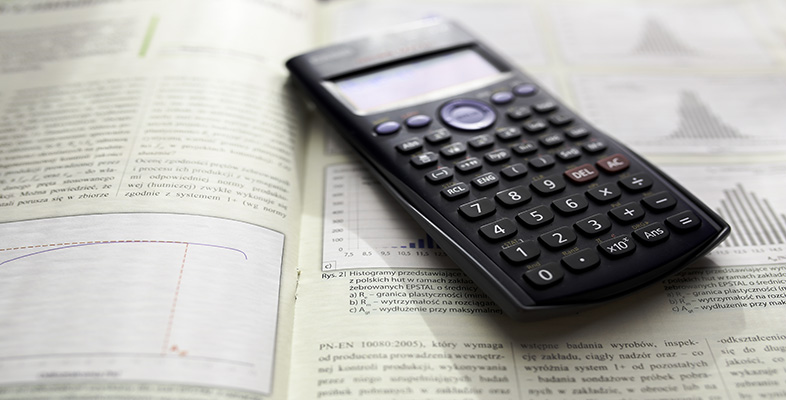Using a scientific calculator

Start this free course now. Just create an account and sign in. Enrol and complete the course for a free statement of participation or digital badge if available.

# 11 Natural logarithms and powers of e on your calculator

Natural logarithms, for example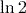, can be evaluated on your calculator using the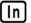key. The second function of this key  (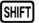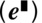) permits the calculation of powers of. Note that an approximate value foritself can be obtained using the key sequence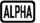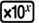().

Remember that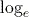means the same as ln. Some calculators have a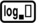key instead of.

## Activity 20 Calculating natural logarithms and powers of e

Calculate the value of each of the following using your calculator, giving each answer to 3 significant figures.

1.2.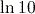3.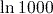4.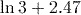5.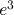1.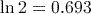(to 3 significant figures).
2.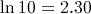(to 3 significant figures).
3.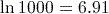(to 3 significant figures).
Notice that this is about three times the answer to part (2). In fact, the exact value ofis precisely three times the exact value of. This is because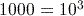, and for any base of logarithm,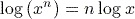. This is an important result for logarithms. In this case we have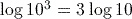.
4.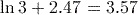(to 3 significant figures).
5.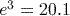(to 3 significant figures).